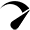# Cap Rate Calculator

Cap rate or capitalization rate is often used to value income property. It is a simple calculation (net income/value=Cap rate), but finding the right numbers to plug into that calculation can be difficult. This calculator is very easy to use, just plug in two of the three numbers and the third number will calculate for you.

If you need help finding that net income number check out our cash flow calculator, but remember Cap rates do not include debt. So if you have a loan make sure to add the loan payments back onto the cash flow number.

This article goes over Cap rates and how they affect the value of properties. Understanding and calculating the Cap Rate.

Video coming soonOptimized by Seraphinite Accelerator